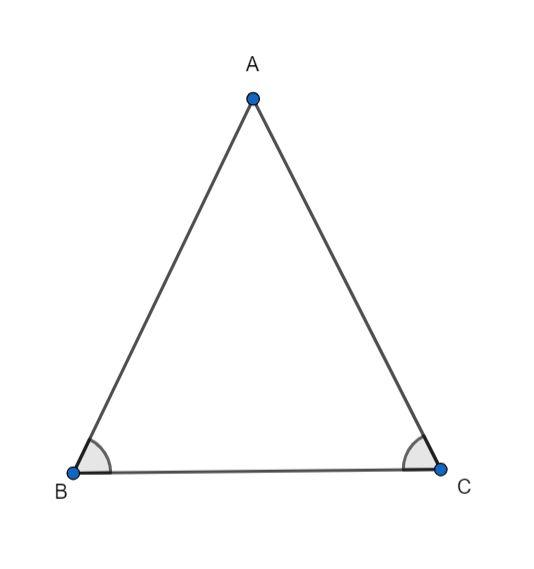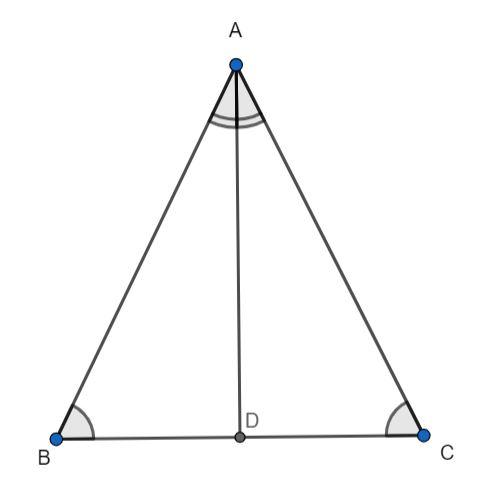Courses
Courses for Kids
Free study material
Offline Centres
MoreLast updated date: 02nd Dec 2023
Total views: 381.9k
Views today: 5.81k

# What is the relation between sides opposite to equal angles?(a) Equal(b) Unequal(c) May or may not be equal(d) None of theseVerified
381.9k+ views
Hint: Construct an angle bisector from the vertex where the sides opposite to equal angles intersect and then using the properties of congruence of triangle, determine the relation between these two sides.Consider triangle ABC, where angle B and angle C are equal. We need to determine the relation between sides AC and AB which are opposite to the angles B and C respectively.
It is given that angle B and angle C are equal, hence, we have:
$\angle ABC = \angle ACB............(1)$
We can determine the relation between sides AB and AC by constructing an angle bisector from vertex A and considering the resulting two triangles.
We draw an angle bisector AD from vertex A to intersect BC at D. Now, we have two triangles, triangle ADB and triangle ADC.We know that angle ABC is equal to angle ABD and angle ACB is equal to angle ACD.
$\angle ABD = \angle ABC...........(2)$
$\angle ACD = \angle ACB...........(3)$
Using equation (2) and (3) in equation (1), we have:
$\angle ABD = \angle ACD...........(4)$
Since, AD is the angular bisector, it divides angle A into equal angles as follows:
$\angle BAD = \angle CAD...........(5)$
The side AD is common to both the triangles. Hence, we have:
$AD = AD...........(6)$
From equations (4), (5) and (6), we observe that the two triangles are congruent by AAS (Angle-Angle-Side) criterion.
$\Delta ABD = \Delta ACD {\text{ (AAS - criterion)}}$
We know that the ratio of the corresponding sides of congruent triangles are equal.
$\dfrac{{AB}}{{AC}} = \dfrac{{BD}}{{CD}} = \dfrac{{AD}}{{AD}}$
Considering the first and the last term, we have:
$\dfrac{{AB}}{{AC}} = \dfrac{{AD}}{{AD}} = 1$
$AB = AC$
Hence, the two sides are equal.
Hence, the correct answer is option (a).

Note: You may make a mistake if you try to construct a median from the vertex joining the sides opposite to equal angles and try to prove congruence between resulting triangles by SSA criterion, which does not exist for proving congruence between two triangles.